# 校訓：信、望、愛

( 林前 13：13 )

Curriculum

 Learning Unit Learning Objective Surds Rationalize the denominators of expressions ofthe form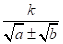. Mathematical Induction Understand the principle of mathematicalinduction. Binomial Theorem Expand binomials with positive integral indicesusing Binomial Theorem. More about Trigonometric Functions Understand the concept of radian measure. Find arc lengths and areas of sectors throughradian measure. Understand the functions cosecant, secant andcotangent and their graphs. Understand the identities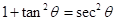and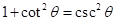. Understandcompound angle formulae and double angle formulae for the functions sine,cosine and tangent, and product-to-sum and sum-to-product formulae for the functionssine and cosine. Introduction to the number e Recognize the definitions and notations of thenumber e and the natural logarithm. Limits Understand the intuitive concept of the limitof a function. Find the limit of a function. Differentiation Understand the concept of the derivative of a function. Understand the addition rule, product rule,quotient rule and chain rule of differentiation. Find the derivatives of functions involvingalgebraic functions, trigonometric functions, exponential functions andlogarithmic functions. Find derivatives by implicit differentiation. Find the second derivative of an explicitfunction. Applications of Differentiation Find the equations of tangents and normal to acurve. Find maxima and minima. Sketch curves of polynomial functions andrational functions. Solve the problems relating to rate of change,maximum and minimum. Indefinite Integration Recognize the concept of indefiniteintegration. Understand the properties of indefinite integrals anduse the integration formulae of algebraic functions, trigonometric functionsand exponential functions to find indefinite integrals. Understand the applications of indefinite integrals in real-lifeor mathematical contexts. Use integration by substitution to find indefiniteintegrals. Use trigonometric substitutions to find the indefinite integralsinvolving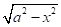,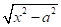or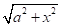. Use integration by parts to find indefinite integrals. Definite Integration Recognize the concept of definite integration. Understand the properties of definite integrals. Find definite integrals of algebraic functions,trigonometric functions and exponential functions. Use integration by substitution to find definiteintegrals. Use integration by parts to find definite integrals. Understand the properties of the definite integrals of even,odd and periodic functions. Applications of Definite Integration Understand the application of definite integrals in findingthe area of a plane figure. Understand the application of definite integrals infinding the volume of a solid of revolution about a coordinate axis or a lineparallel to a coordinate axis. Determinants Recognize the concept and properties of determinants oforder 2 and order 3. Matrices Understand the concept, operations and properties of matrices. Understand the concept, operations and properties of inversesof square matrices of order 2 and order 3. Systems of Linear Equations Solve the systems of linear equations of order 2 and order3 by Cramer’s rule, inverse matrices and Gaussian elimination. Introduction to Vectors Understand the concepts of vectors and scalars. Understand the operations and properties of vectors. Understand the representation of a vector in the rectangularcoordinate system. Scalar Product and Vector Product Understand the definition and properties of the scalar product(dot product) of vectors. Understand the definition and properties of the vector product(cross product) of vectors in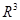. Applications of Vectors Understand the applications of vectors. Inquiry and Investigation Through various learning activities, discover andconstruct knowledge, further improve the ability to inquire, communicate,reason and conceptualize mathematical concepts.